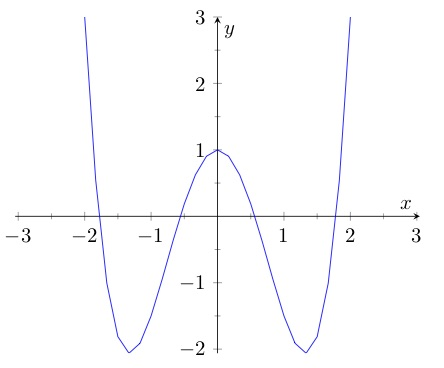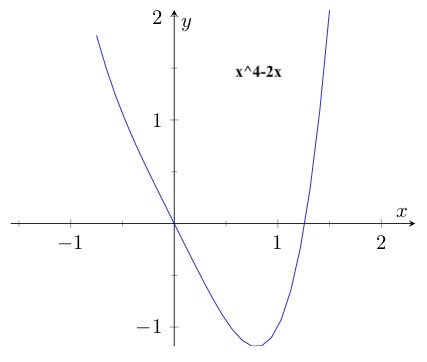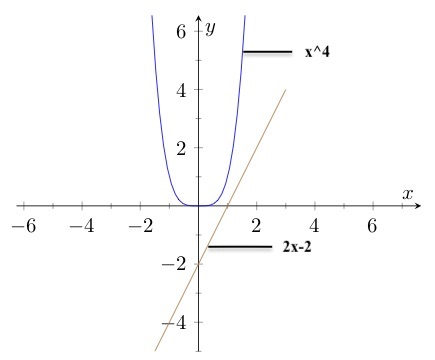# How Many x-Intercepts?

## Question

What is the maximum number of zeros (also called x-intercepts) the function    may have? What is the minimum? c, a constant, may be any real number.

## Solution

The maximum number zeros of a polynomial function is equal to the function’s degree. The function  is fourth degree, so it may have up to four zeros. A fourth-degree function may look like this:Four is the max. Will the function  necessarily have four? Let’s try to narrow down the possibilities.

Try a graph. You can graph this function if you assign a value to c. Let’s try , so that the function is . That graphs up like this:That gives you two x-intercepts. but with a different value of c could this function may have more x-intercepts — or fewer?

At the x-intercepts, the y-value is 0:

That means

That means that for this function, at the zeros, the fourth-degree monomial   equals the linear function . Let’s graph these two pieces separately for  , the value of c that we’ve seen gives two x-intercepts.These two pieces intersect in to placesone for each of the two zeros. Are any other points of intersection are possible? On the left-hand side, the fourth-degree term just keeps going up while the line just keeps going down, so there are no more points of intersection on the left. On the right-hand side to the right of both points of intersection, the fourth-degree term is always steeper than the line and is therefore always greater, so there are no more points of intersection on the right. Remember that each point of intersection on this graph corresponds to a zero on the function , so that means no other zero is possible for the function.

But that doesn’t cover all possibilities, because we considered only one value for c. What if c takes a different value? Then the line slides up or down. If  , the line slides up and there will two points of intersection, one at which x is less than zero and one in which x is greater than zero.

Slide it down just a little from and there are still two points of intersection, this time both positive. Slide it farther and eventually it will not intersect the fourth-degree curve at all.For even higher values of c – meaning the line slides farther down on the y-axis – the function has no zeros.

So for the function , the minimum number of x-intercepts is zero and the maximum number is two.

# Vertical Asymptotes

Here’s a video about vertical asymptotes. In rational functions, these can happen when a denominator hits zero.

Questions?

Related: Last time’s video about horizontal asymptotes and next time’s video about oblique (aka slant) asymptotes.

# Horizontal Asymptotes

Here’s a video about horizontal asymptotes. In rational functions, these happen when the numerator’s degree equals the denominator’s and when numerator’s degree is less than the denominator’s.

Questions?

Videos about vertical and oblique (aka slant) asymptotes will follow in the coming weeks.